Rocco 等人在其弱监督语义级别图像匹配的工作中，将特征匹配与随机采样一致性算法（RANdom SAmple Consensus, RANSAC）联系在一起，提出了一个可微分的基于语义的评分损失函数，文中对于语义特征匹配和 RANSAC 算法的阐述令人耳目一新，遂作此文对相关概念追本溯源。

## 随机采样一致性（RANSAC）

1. 从数据样本 $S$随机选取 $K$ 个点，得到假设子集 $S_K$，然后使用 $S_K$ 优化模型参数 $M(\theta)$，得到初始模型；
2. 对所有数据样本 $S$ 应用模型 $M(\theta)$，然后根据每个数据样本的误差 $||M(\theta, x_i) - y_i||$ 来所有数据样本划分为内群点（inliner points）和离群点（outliner points），其中误差大于超参数 $\epsilon$ 的称为离群点 $S_{outliner}$，反正则为内群点 $S_{inliner}$
3. 如果内群点的占比 $R = \frac{S_{inliner}}{S}$ 大于超参数阈值 $\tau$，则使用所有的内群点 $S_{inliner}$ 来重新优化模型参数，并将得到的模型作为最终模型，终止迭代；
4. 否则使用内群点 $S_{inliner}$ 优化模型，并重复执行步骤 2，直到条件 3 满足，或者达到迭代次数上限 $N$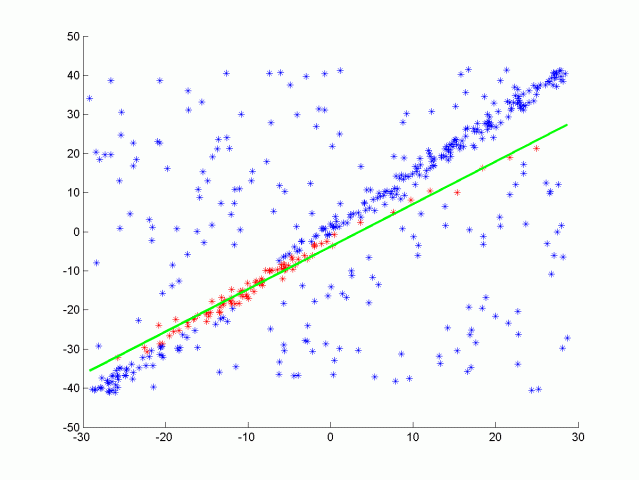$1 - p = (1 - u^K)^N$

$N = \frac{log(1 - p)}{log(1 - (1 - v)^K)}$

## 图像特征匹配（Image Feature Matching）

1. 从图像对 $(I_s, I_t)$ 中分别提取特征点 $(F_s, F_t)$
2. 对两个特征点集进行匹配，得到匹配完毕的特征对 $M_{s \rightarrow t}$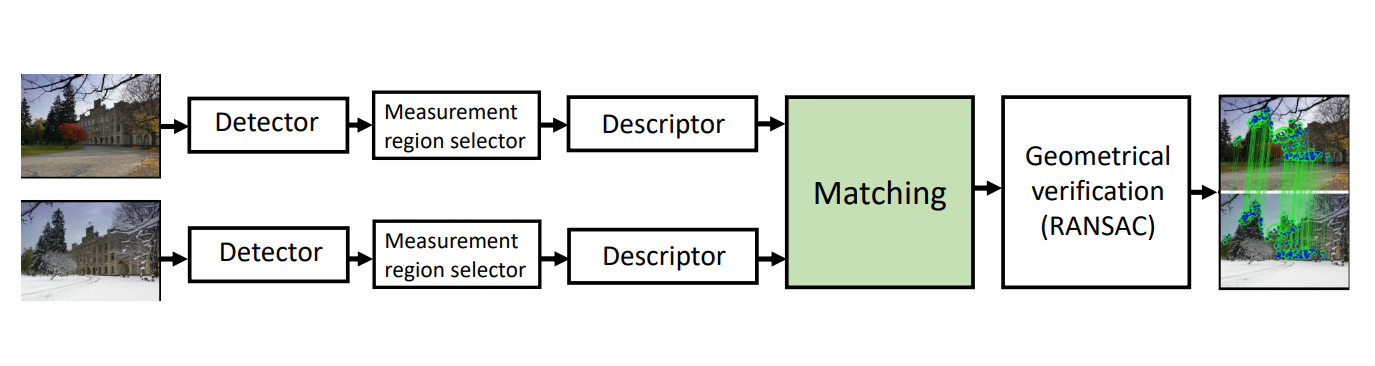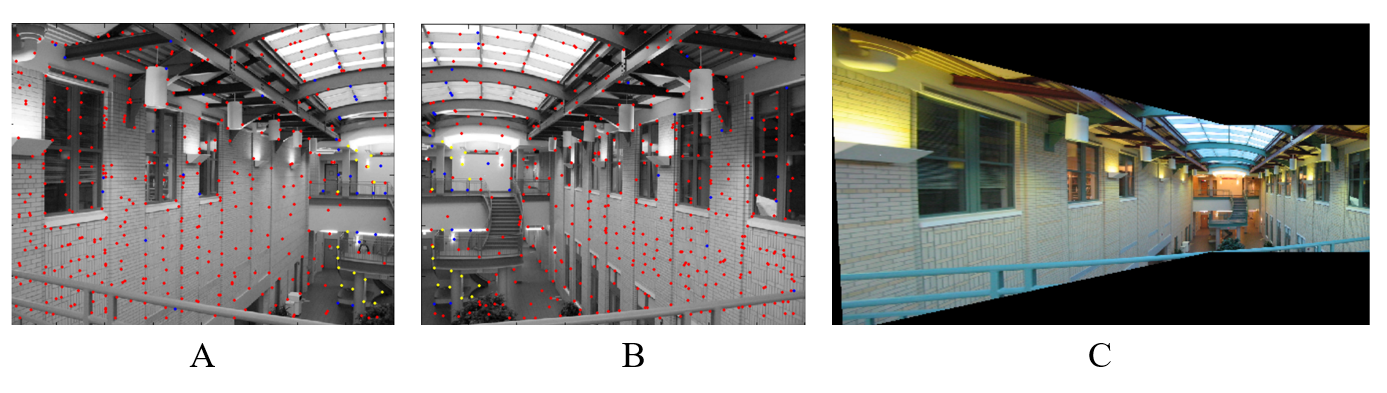1. $M_{s \rightarrow t}$ 中随机选取 $k$ 个特征点对 $M_k$
2. 使用 $M_k$ 求解单应矩阵 $H$
3. 对图像 $I_s$ 中的所有特征点 $F_s$ 应用单应矩阵 $H$，将其投影到图像 $I_s$ 的成像坐标系中，得到投影后的特征点 $\hat F_s = Project(H, F_s)$
4. 根据预设定阈值 $\epsilon$ 计算内群点，即满足 $D(\hat{F_s}, M_{s \rightarrow t}F_s) \leq \epsilon$ 的匹配；
5. 如果内群点占比不满足条件，则重复上述步骤 2 - 4；否则使用当前的所有内群点对重新计算 $H$ 作为最终结果。

## 语义特征匹配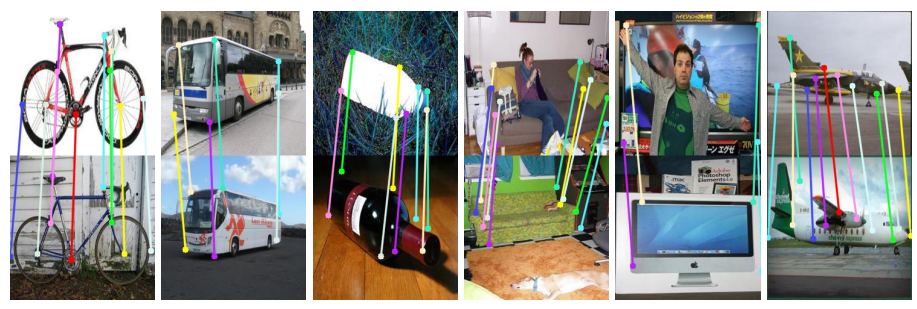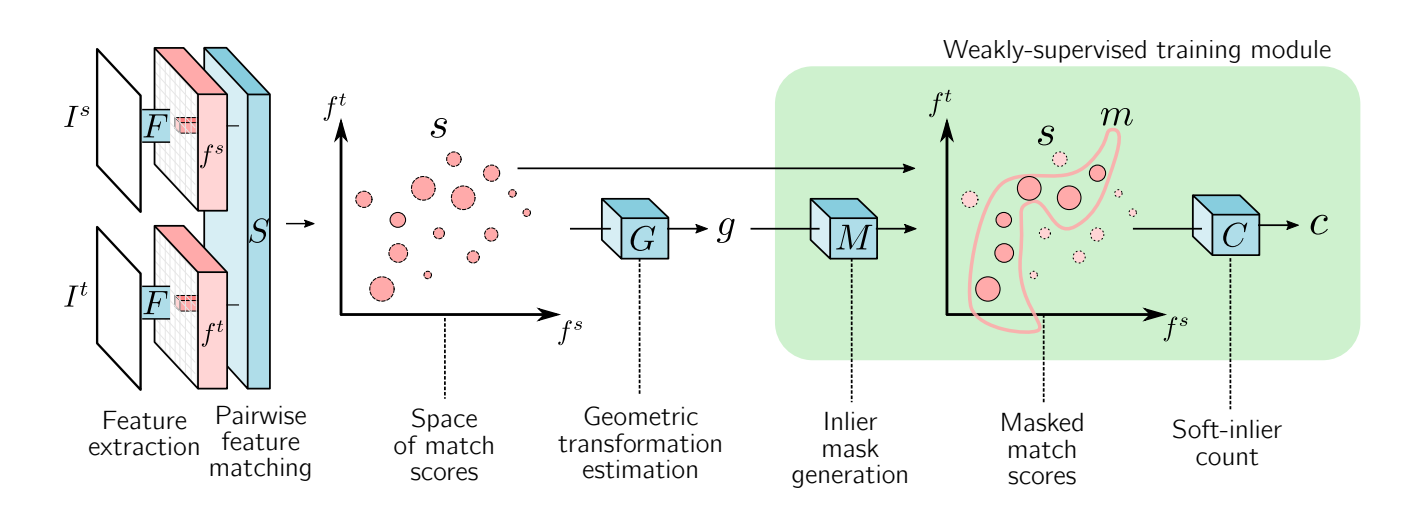$V_s = Flatten(F_s), V_t = Flatten(F_t); R^{h \times w \times c} \rightarrow R^{hw \times c}$

$C = Softmax(V_sV_t^T); R^{hw \times c} \times R^{c \times hw} \rightarrow R^{hw \times hw}$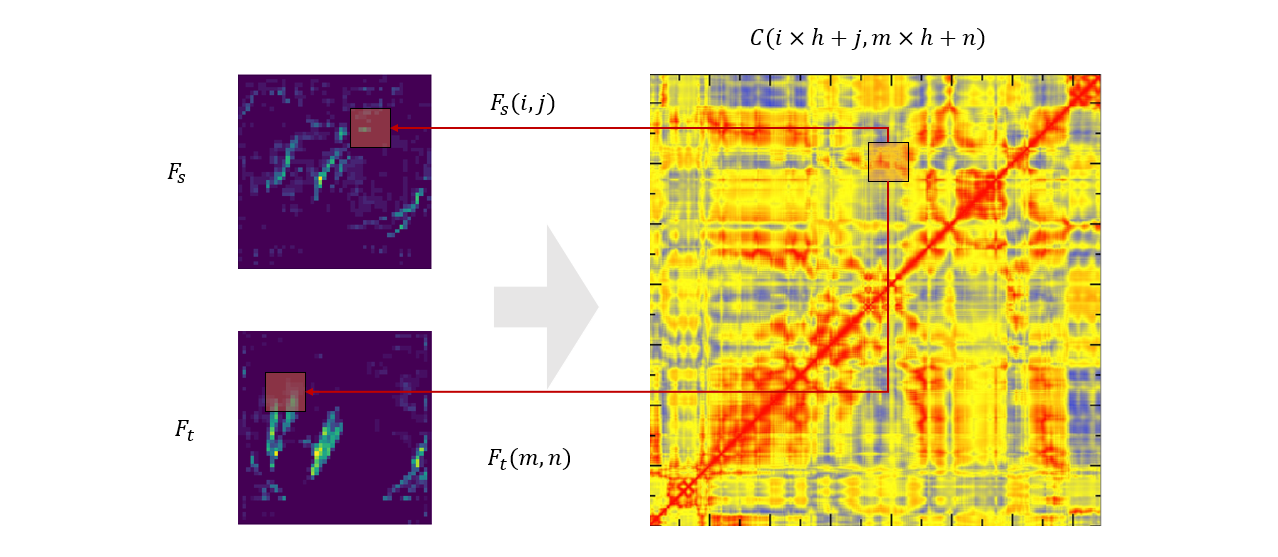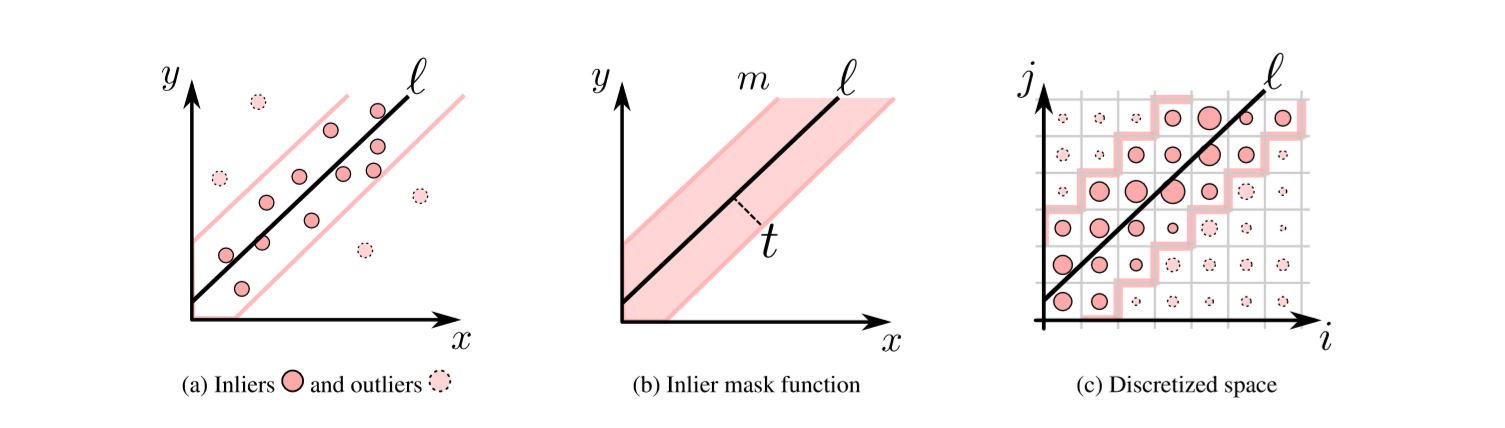## 参考资料

1. Rocco, Ignacio, Relja Arandjelović, and Josef Sivic. "End-to-end weakly-supervised semantic alignment." Proceedings of the IEEE Conference on Computer Vision and Pattern Recognition. 2018.
2. Derpanis, Konstantinos G. "Overview of the RANSAC Algorithm." Image Rochester NY 4.1 (2010): 2-3.
3. Fischler, Martin A., and Robert C. Bolles. "Random sample consensus: a paradigm for model fitting with applications to image analysis and automated cartography." Communications of the ACM 24.6 (1981): 381-395.Communications of the ACM, 24(6):381–395, 1981.
4. Torr, Philip HS, and Andrew Zisserman. "MLESAC: A new robust estimator with application to estimating image geometry." Computer vision and image understanding 78.1 (2000): 138-156.
5. Torr, Philip H. S., and Colin Davidson. "IMPSAC: Synthesis of importance sampling and random sample consensus." IEEE Transactions on Pattern Analysis and Machine Intelligence 25.3 (2003): 354-364.
6. Jin, Yuhe, et al. "Image Matching across Wide Baselines: From Paper to Practice." arXiv preprint arXiv:2003.01587 (2020).
7. Yi, Kwang Moo, et al. "Lift: Learned invariant feature transform." European Conference on Computer Vision. Springer, Cham, 2016.
8. Noh, Hyeonwoo, et al. "Large-scale image retrieval with attentive deep local features." Proceedings of the IEEE international conference on computer vision. 2017.
9. Dusmanu, Mihai, et al. "D2-Net: A Trainable CNN for Joint Description and Detection of Local Features." Proceedings of the IEEE Conference on Computer Vision and Pattern Recognition. 2019.